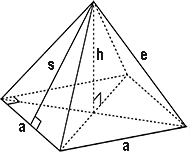# Square Pyramid Calculator

A Square Pyramid can be referred to as a three-dimensional geometric figure that has a square base and four triangular sides that all link in one point. To put it more simply, it is a pyramid with a square base. Interestingly enough, the Pyramid of Giza represents a Square Pyramid.

The sides of a triangular pyramid are all triangles. The axis runs through the centre of the pyramid and is at right angle to its base.

Square Pyramid CalculatorYou may set the number of decimal places in the online calculator. By default there are only two decimal places.

Results
Lateral Surface Area (L):  square units
Base Surface Area (B):  square units
Total Surface Area (A):  square units
Volume (V):  cubic units

### The online Square Pyramid Calculator is apt of solving problems that involve the use of the following formulae:

Slant Height (s) = √(h2 + (1/4) a2)

Lateral surface area (L) = a √(a2 + 4h2)

Surface area of base (B) = a2

Total Area = L + B

Volume = (1/3)a2h